Next: Dimensionless Numbers in Compressible Up: Mathematical Models of Fluid Previous: Equations of Compressible Fluid

# Dimensionless Numbers in Incompressible Flow

It is helpful to normalize the equations of incompressible fluid flow, (1.81)-(1.82), in the following manner: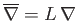,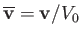,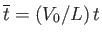,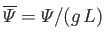, and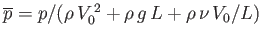. Here,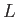is a typical spatial variation lengthscale,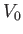a typical fluid velocity, and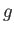a typical gravitational acceleration (assuming thatrepresents a gravitational potential energy per unit mass). All barred quantities are dimensionless, and are designed to be comparable with unity. The normalized equations of incompressible fluid flow take the form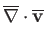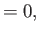(1.90)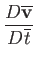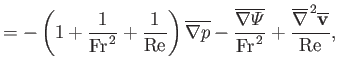(1.91)

where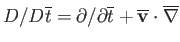, and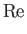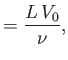(1.92)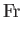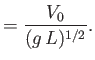(1.93)

Here, the dimensionless quantities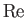and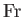are known as the Reynolds number and the Froude number, respectively. [After Osborne Reynolds (1842-1912) and William Froude (1810-1879), respectively.] The Reynolds number is the typical ratio of inertial to viscous forces within the fluid, whereas the square of the Froude number is the typical ratio of inertial to gravitational forces. Thus, viscosity is relatively important compared to inertia when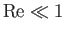, and vice versa. Likewise, gravity is relatively important compared to inertia when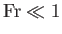, and vice versa. Note that, in principal,andare the only quantities in Equations (1.90) and (1.91) that can be significantly greater or smaller than unity.

For the case of water at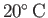, located on the surface of the Earth (Batchelor 2000),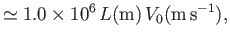(1.94)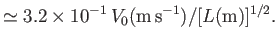(1.95)

Thus, if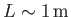and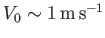, as is often the case for terrestrial water dynamics, then the previous expressions suggest that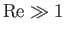and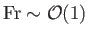. In this situation, the viscous term on the right-hand side of Equation (1.91) becomes negligible, and the (unnormalized) incompressible fluid flow equations reduce to the following inviscid, incompressible, fluid flow equations: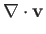(1.96)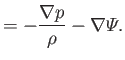(1.97)

For the case of lubrication oil at, located on the surface of the Earth,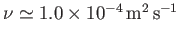(i.e., oil is about 100 times more viscous than water), and so (Batchelor 2000)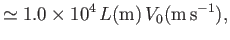(1.98)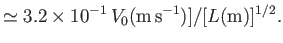(1.99)

Suppose that oil is slowly flowing down a narrow lubrication channel such that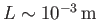and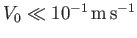. It follows, from the previous expressions, thatand. In this situation, the inertial term on the left-hand side of (1.91) becomes negligible, and the (unnormalized) incompressible fluid flow equations reduce to the following inertia-free, incompressible, fluid flow equations:(1.100)(1.101)Next: Dimensionless Numbers in Compressible Up: Mathematical Models of Fluid Previous: Equations of Compressible Fluid
Richard Fitzpatrick 2016-03-31